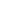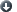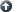## Archived Page

This page has been archived and is provided for historical reference purposes only. The content and links are no longer maintained and may now be outdated.

### Guidelines for Great PresentationsIntroductionBring Your Presentation to LifeCurriculum TopicsTeacher CritiqueStudent CritiqueReferences#### Curriculum Topics Covered in High School Science Courses

The following is a list of curriculum topics covered in selected High School science courses.Biology ABiology BAnatomy and PhysiologyChemistry AChemistry BPhysics APhysics B

#### Biology A

The first semester usually includes study of cell biology, reproduction, genetics, evolution, and plant and animal classifications. Students should be able to:

1. Use methods of qualitative and quantitative observation.
2. Describe the general structures, functions, biochemistry and diversity of cells.
3. Describe levels of organization.
4. Explain perpetuation of species.
5. Apply laws of classical genetics and the principles of chromosomal inheritance to problems of genetic differences in individuals.
6. Explain the general functions of DNA and RNA.
7. Compare scientific theories of the origin and evolution of living things.
8. Apply methods of taxonomy to classify organism.
9. Identify career opportunities in the biological area.Top of page

#### Biology B

The study of ecology, behavior, and human structure and function are covered.
Students should be able to:

1. Describe the characteristics of microorganisms.
2. Describe general anatomy and physiology of plant and animals.
3. Explain the biological behavior of living things.
4. Understand the relationships in energy flow patterns, and the development of the ecosystem.
5. Analyze the skills required for the practice of biotechnology.

Students usually have had an introductory course in chemistry and biology. Students should be able to:

1. Explain the phenomena of free energy change and entropy.
2. Trace the history of cytology.
3. Relate the C3, C4, and CAM pathway to plant anatomy, photosynthesis and the environment.
4. Describe the models for gene regulation.
5. Understand genetic abnormalities with emphasis on chromosomal aberrations.
6. Discuss the structure, physiology, reproduction, pathology and economic importance of viruses and Monerans.
7. Trace various immunological responses.
8. Relate the Hardy -Weinberg law, genetic drift, and balanced poly-morphism to evolutionary situations.
9. Interpret current issues in biology, including population growth, ecological intervention, and biomedical progress.Top of page

#### Anatomy and Physiology

This course is intended for students who have had general biology and may have had chemistry. Students study cells, their sub-microscopic parts and how their structure relates to function. They study the major organ systems of the human body: skeletal, muscular, nervous, and digestive system.Top of page

#### Chemistry A

Chemistry A topics include the organization and classification of matter, atomic theory, radioactivity, the periodic table of the elements, principles of chemical reactions, heat, molecular motion, and chemical bonds. Students should be able to:

1. Differentiate among elements, compounds, and mixtures, using physical and chemical properties.
2. Distinguish among physical, chemical, and nuclear changes.
3. Select and use mathematical relationships and computational skills.
4. Perform laboratory skills appropriate to chemistry.
5. Use chemical symbols to write formulas and name compounds.
6. Describe the sequence of selected discoveries, which resulted in modern atomic theory.
7. Predict physical and chemical properties based on the periodicity of the elements.
8. Imply the mole concept operationally and conceptually.
9. Construct chemical equations.
10. Solve problems involving quantitative relationships in equations.
11. Relate the state and energy content of a substance to the degree of motion in its molecules.
12. Apply the gas laws.
13. Account for the attractions among particles and the effect of these forces on the properties of the resulting substances.Top of page

#### Chemistry B

Chemistry B topics include energy, chemical reactions, equilibrium reactions, chemical solutions, acids, bases, salts, electrochemistry, and carbon and its compounds. Students should be able to:

1. Determine the amount of energy involved in a reaction.
2. Determine the driving forces of a reaction.
3. Interpret the practical implications of the laws of thermodynamics.
4. Describe the factors that affect the rates of reactions.
5. Describe systems of equilibrium.
6. Calculate the quantities needed to prepare and use solutions employing various units for expressing concentrations.
7. Analyze factors that determine the properties of solutions.
8. Identify reactions involving ions in aqueous solutions.
9. Analyze the role of acids, bases and salts and their interactions.
10. Apply electrochemical principles.
11. Balance oxidation-reduction equations.
12. Apply principles of oxidation and reduction.
13. Classify carbon compounds.
14. Illustrate the significance of carbon and its compounds.Top of page

#### Physics A

This course treats vector analysis, the study of motion (kinematics), the effect of force on moving bodies (dynamics), energy and momentum. Student should be able to:

1. Apply vectors to the solution of physics problems.
2. Analyze rectilinear motion.
3. Analyze curvilinear motion.
4. Apply Newton's laws of motions.
5. Develop the law of universal gravitation.
6. Apply the law of conservation of mass-energy.
7. Apply the law of conservation of momentum to the interaction of objects.Top of page

#### Physics B

Physics B covers the topics of thermodynamics-the effect of heat on motion, electricity, magnetism, wave motion, and modern physics. Student should be able to:

1. Solve problems of thermodynamics.
2. Describe the phenomena related to electrostatic charge.
3. Apply Coulomb's law.
4. Apply principles of electrostatic potential and potential difference.
5. Solve problems involving electrical circuits.
6. Solve problems involving magnetism.
7. Analyze the behavior of waves.
8. Describe models of the atom.
9. Describe the dual nature of light and matter.

If there is a particular topic the scientist would like the students to be aware of, the teacher can be notified. Pertinent pamphlets, handouts, a film (if available), or other preparation can be provided several days before the presentation.Top of page

Last updated: May 01, 2006